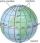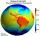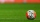# Perimeter + sphere - math problems

#### Number of problems found: 6

• The EarthThe Earth's surface is 510,000,000 km2. Calculates the radius, equator length, and volume of the Earth, assuming the Earth has the shape of a sphere.
• Earth's circumferenceCalculate the Earth's circumference of the parallel 48 degrees and 10 minutes.
• Earth parallelEarth's radius is 6370 km long. Calculate the length parallel of latitude 50°.
• Average speedWhat is the average speed you have to move the way around the world in 80 days? (Path along the equator, round to km/h).
• Rotation of the EarthCalculate the circumferential speed of the Earth's surface at a latitude of 61°​​. Consider a globe with a radius of 6378 km.
• What is bigger?Which ball has a larger volume: a football with a circumference of 66 cm or a volleyball with a diameter of 20 cm?

We apologize, but in this category are not a lot of examples.
Do you have an interesting mathematical word problem that you can't solve it? Submit a math problem, and we can try to solve it.

We will send a solution to your e-mail address. Solved examples are also published here. Please enter the e-mail correctly and check whether you don't have a full mailbox.

Please do not submit problems from current active competitions such as Mathematical Olympiad, correspondence seminars etc...

Perimeter - math word problems. Sphere Problems.